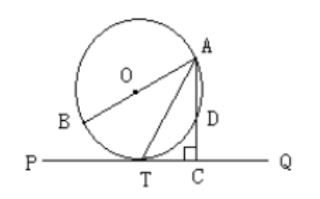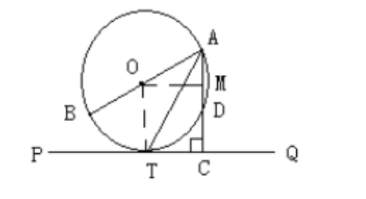（1）求证: $\mathrm{AT}$ 平分 $\angle B A C$ 。
(2) 若 $\mathrm{AD}=2, T C=\sqrt{3}$, 求 $\odot O$ 的半径。(1) 证明： 连接 OT,
$\because \mathrm{PQ}$ 切 $\odot O$ 于 $\mathrm{T}$,
$$\therefore O T \perp P Q$$
$$\text { 又 } \because A C \perp P Q, \therefore O T \| A C$$
$$\therefore \angle T A C=\angle A T O$$
$$\text { 又 } \because 0 \mathrm{~T}=0 \mathrm{~A}$$
$$\therefore \angle A T O=\angle O A T \text {, }$$
$\therefore \angle O A T=\angle T A C$, 即 AT 平分 $\angle B A C$ 。(2) 过点 $\mathrm{O}$ 作 $O M \perp A C$ 于 $\mathrm{M}$,
$$\therefore A M=M D=\frac{A D}{2}=1 \text {, }$$
$$\text { 又 } \angle O T C=\angle A C T=\angle O M C=90^{\circ}$$
$\therefore$ 四边形 $0 T C M$ 为矩形
$$\therefore O M=T C=\sqrt{3}$$
$\therefore$ 在 $R t_{\triangle} A O M$ 中, $A O=\sqrt{O M^2+A M^2}=\sqrt{3+1}=2$, 即 $\odot O$ 的半径为 2 。
①点击 收藏 此题， 扫码注册关注公众号接收信息推送（一月四份试卷,中1+高2+研1）
② 程序开发、服务器资源都需要大量的钱，如果你感觉本站好或者受到到帮助，欢迎赞助本站,赞助方式：微信/支付宝转账到 18155261033

 ①此题难易度如何 ②此题推荐度如何 确定